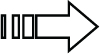# CHAPTER 10Using Formulas for Common Mathematical Operations

Most Excel analysts working in the corporate world will be asked to perform mathematical operations that provide insight into key operational metrics. In this chapter, you'll get to explore a few mathematical operations commonly used in the world of business analytics.

## Calculating Percentages

Calculations such as percent of totals, variance to budget, and running totals are the cornerstone of any basic business analysis. In this section, you'll explore some examples of formulas that will help with these types of analyses.This book's website, `www.wiley.com/go/excel2019bible`, includes a copy of the sample workbook for this chapter. The file is named `Mathematical Formulas.xlsx`.

### Calculating percent of goal

When someone asks you to calculate a percent of goal, they are simply asking you to compare the actual performance against a stated goal. The math involved in this calculation is simple: divide the actual by the goal. This will give you a percentage value that represents how much of the goal has been achieved. For instance, if your goal is to sell 100 widgets and you sell 80 widgets, your percent of goal is 80% (80/100).

Figure 10.1 shows a list of regions with a column for goals and a column for actuals. Note that the formula ...

Get Excel 2019 Bible now with the O’Reilly learning platform.

O’Reilly members experience books, live events, courses curated by job role, and more from O’Reilly and nearly 200 top publishers.